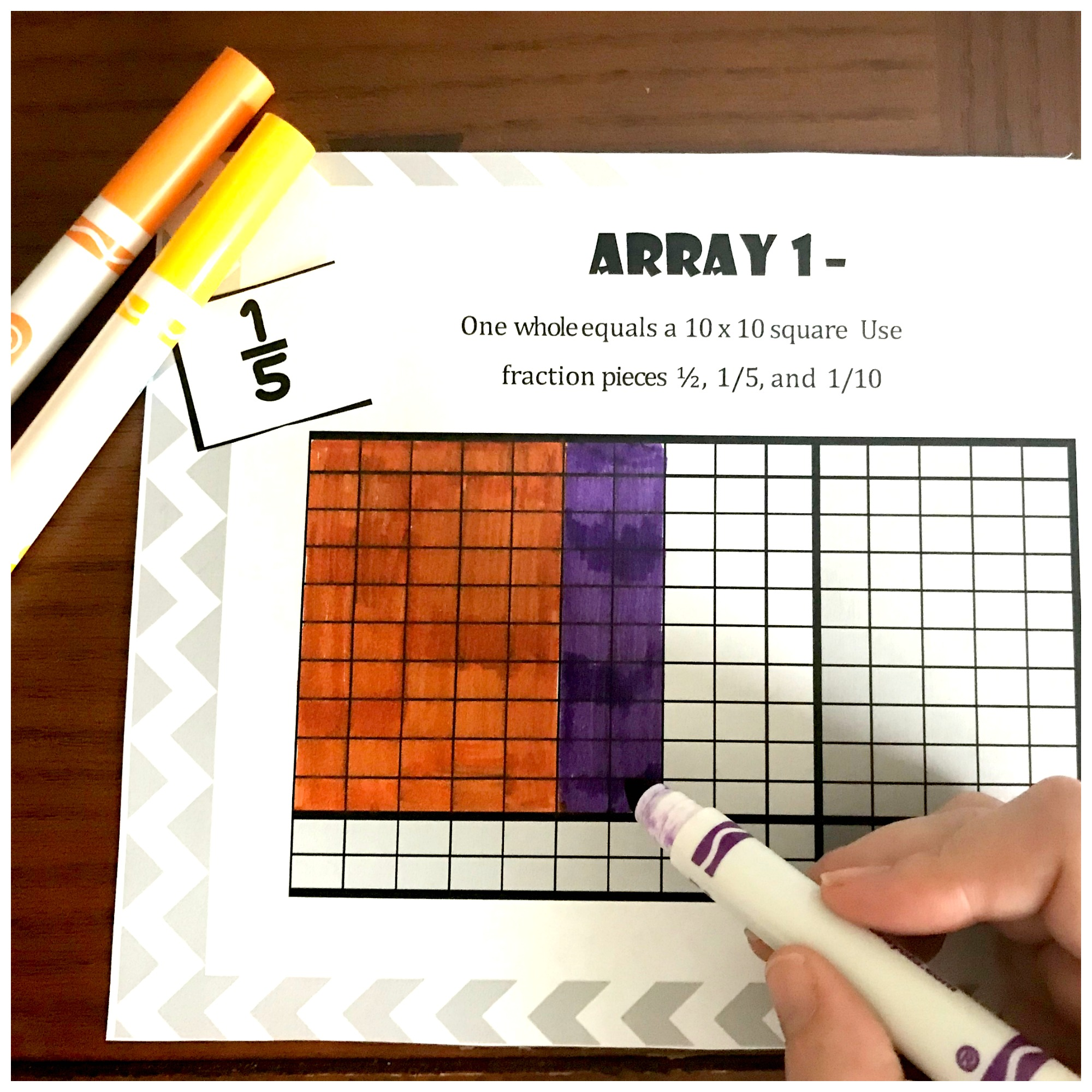# Multiplication Arrays Games | Free Printable

## Multiplication Arrays Games

One of the best ways to help kids learn multiplication is through fun games! These multiplication arrays games will help kids learn how to multiply fractions with arrays by visualizing and understanding how multiplication works.

This is a great game for teachers and moms to try out with their kids!

## Multiplying Fraction with Arrays: A Better Method

When you were a fifth-grade student sitting at your desk, you probably did this concept totally different than it is done today.

You took your mixed numbers, changed them to improper fractions, multiplied them together, and then changed that large number back into a mixed number.

It was a lot of steps, often with large numbers, and mistakes were easy to make. If your understanding of fractions was anything similar to mine, you didn’t have a clue why you were doing those steps.

## Using Arrays to Multiply Fractions

Nowadays, using a visual model has become the keyword, and I love it. I’m not saying the steps are shorter, but the students are not just doing random steps.

They are visualizing the problem, and through that, they are understanding the WHY behind the steps. I, of course, love that.

If you are looking for more information on how to multiply fractions by fractions….this is a great place to start.

## How to Multiply Fractions With Arrays

I will walk you through how to teach your students how to multiply fractions using arrays. It is easy. Take a look at this example.

As you can see we are multiplying 2 1/4 by 1 1/3.

To begin, we draw three wholes across at the top (you will always round up your fraction to the next whole when drawing an array).

Then color in the first two wholes, and then color in 1/4 of the third whole.

Next, we need to have two wholes going down. One for the 1 and another whole for the 1/3. One whole is already there so I only need to draw one more whole.

We all know that an array is a rectangle, so I need to finish drawing wholes horizontally until I have a complete rectangle. In this example, I would only have to draw one more.

Now it is time to color it in. Next, we need to color in 1 1/4 vertically. The trick here is to realize that one whole is already colored in so all we have to do is color in 1/3.

Finally, it is important at this point to extend the 1/4 to the bottom of the area and the 1/3 all the way across. Notice that this divides the box into twelfths.

Isn’t that an awesome representation of why we multiply the denominator?

Math time: Now we get to add up the colored in part of the array: 2 +1/3 + 1/3 + 1/4 + 1/12 = 3

## The Old Fashion Way

Of course, you can also have them multiply the numbers like they have done in third and fourth grade when multiplication was taught.

2 x 1 = 2

2 x 1/3= 2/3

1/4 x 1 = 1/4

1/4 x 1/3 = 1/2

2 + 2/3 + 1/4 + 1/12 = 2

The arrays may be a little confusing at first for our precious students. They need time playing with them, and being able to recognize what they are seeing.

## Free Multiplication Array Game

I created this FREE multiplying mixed numbers game to help students get practice playing with arrays, learn to see the fractional parts in them, and ultimately be able to multiply fractions with arrays.

## Interactive Arrays for Multiplication

1. Print off enough arrays for each child to have their own.
2. Laminate the boards or place them in sheet protectors.
3. Print off the game pieces and cut them off.
4. Provide dry erase markers.

## Fraction Arrays: How to Play!

I highly recommend playing this game in a small group at the beginning. This FREEBIE has detailed directions, discussion questions, and variations to the game, but is more manageable in a small group setting.

1. Player one draws a fraction card and colors in that fraction based on what 1 equals in the array. The first square in the array equals 1 whole. (Example: If the square is a 10×10 and the player draws ½ then the player will color in 50 small squares or 5 rows.) When finished, the player put the fraction face down at the bottom of the pile.
2. Player two then draws a card and colors in that fraction based on what 1 equals in the array.
3. The players continue to take a turn. Whoever fills up the whole array first, wins.
4. To make the game more challenging you could have the children fill up the array exactly, or if you go over you have to erase that amount instead of add.

On the directions, there are some great questions to help you engage your children and help them make connections to multiplications!

Games are a fun way to help children experience and begin to love math. I hope that this game cultivates a love for math in your kiddos.

## Multiplication Array Games Printable

Below is the multiplication arrays game that teaches students how to multiply fractions with arrays. You can also try this for more practice, Decimal Multiplication With Arrays and Lattice Work.

color-an-array-4